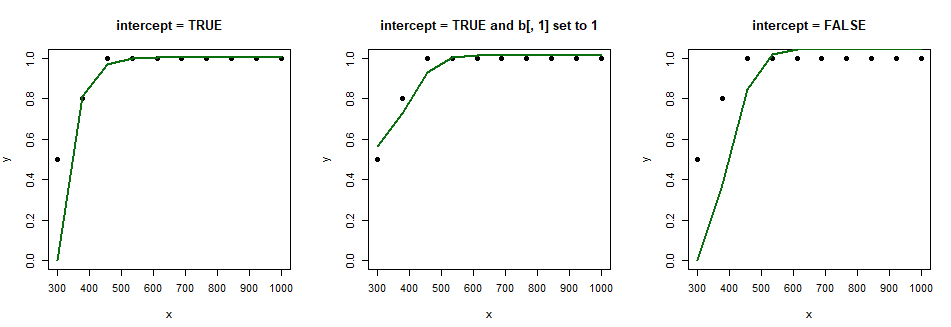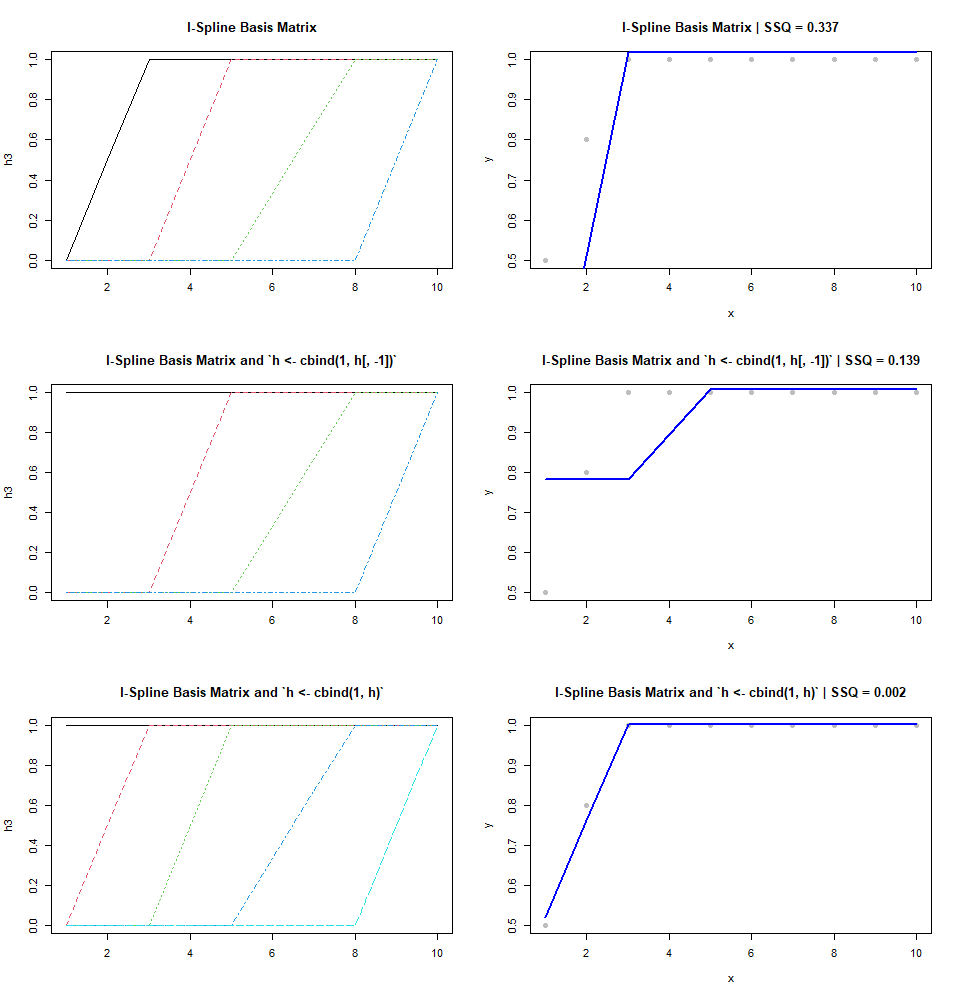# What is the role of an intercept in an I-Spline basis?

I have the following data to which I want to fit a monotone non-decreasing spline.

x <- c(300, 377, 455, 533, 611, 688, 766, 844, 922, 1000)
y <- c(0.5, 0.8, 1, 1, 1, 1, 1, 1, 1, 1)


To achieve this, I use an I-Spline basis (i.e., splines2::iSpline) paired with non-negative coefficients (i.e., nnls::nnls).

# Inner knots.
k <- quantile(x, probs = c(.25, .5, .75))

h <- splines2::iSpline(x, knots = k, Boundary.knots = range(x), intercept = TRUE)
a <- nnls::nnls(h, y)\$x


And this gives me the following:

# Fitted.
f <- h %*% a

print(f)
#             [,1]
#   [1,] 0.0000000
#   [2,] 0.8106201
#   [3,] 0.9699174
#   [4,] 0.9992354
#   [5,] 1.0035000
#   [6,] 1.0035515
#   [7,] 1.0035515
#   [8,] 1.0035515
#   [9,] 1.0035515
#  [10,] 1.0035515


However, with intercept = TRUE, I would expect the spline to start somewhere above the first data point. For instance, this seems to be the case when I set the first basis function to 1.

h[, 1] <- 1


If I set intercept = FALSE, then things go haywire.Can you please help me understand what is going on, i.e., why is the spline not starting at (300, 0.5)?

### Further Details

I want to clarify my question further. My confusion stems from what is written in this paper on p. 15:

To get a basis for the increasing splines we need to add the constant function to the I-splines and allow it to enter the linear combination with either sign.

In the splines2 package documentation, where the intercept argument for iSpline() is described, it says:

Notice that when using I-Spline for monotonic regression, intercept = TRUE should be set even when an intercept term is considered additional to the spline bases in the model.

What I get from this is that if I want to fit a monotone spline with, say, nnls::nnls(), then I need to add a constant function to the I-Spline basis, i.e., h <- cbind(1, h), assuming that h is the basis matrix output of iSpline().

However, in the paper linked above, that's not always the case. Sometimes the author replaces the first column of the basis matrix (i.e., h <- cbind(1, h[ -1]) with the constant function, and, other times, he appends the constant function to the basis matrix.

For instance, if I create the I-Spline basis matrix as follows:

h <- splines2::iSpline(x, knots = c(3, 5, 8), degree = 0, intercept = TRUE)


Then, depending on how I "add" the constant function (or not) I get different splines:What's not clear to me is how the basis matrix provided by iSpline() is intended to be used in this case.

From the definition of k in your code we know the first segment is fitting more than 2 data points.

Only if it is fitting first 2 data points and without the constraint that the function to be continuous, the spline will pass the first point. Note, if that is the case, the model is not a spline.

library(ggplot2)
x <- c(300, 377, 455, 533, 611, 688, 766, 844, 922, 1000)
y <- c(0.5, 0.8, 1, 1, 1, 1, 1, 1, 1, 1)
group <- c(0,0,1, 1, 1, 1, 1, 1, 1, 1)
d = data.frame(x=x,y=y, group=group)
ggplot(d,aes(x,y, group=group))+geom_point()+geom_smooth(method='lm')+theme_gray(20)• Haitao Du, what you wrote makes sense. However, I am still confused because in this paper on p. 15 (last sentence), it says that a constant function needs to be added to the I-Spline basis matrix. However, in the paper, the first column of the basis matrix is sometimes replaced with ones (i.e., h <- cbind(1, h[ -1]) and other times simply another column is appended (i.e., h <- cbind(1, h)). Can you please shed some light on why this is the case? – Mihai Apr 20 at 8:59
• I will append this to the question above. – Mihai Apr 20 at 9:15